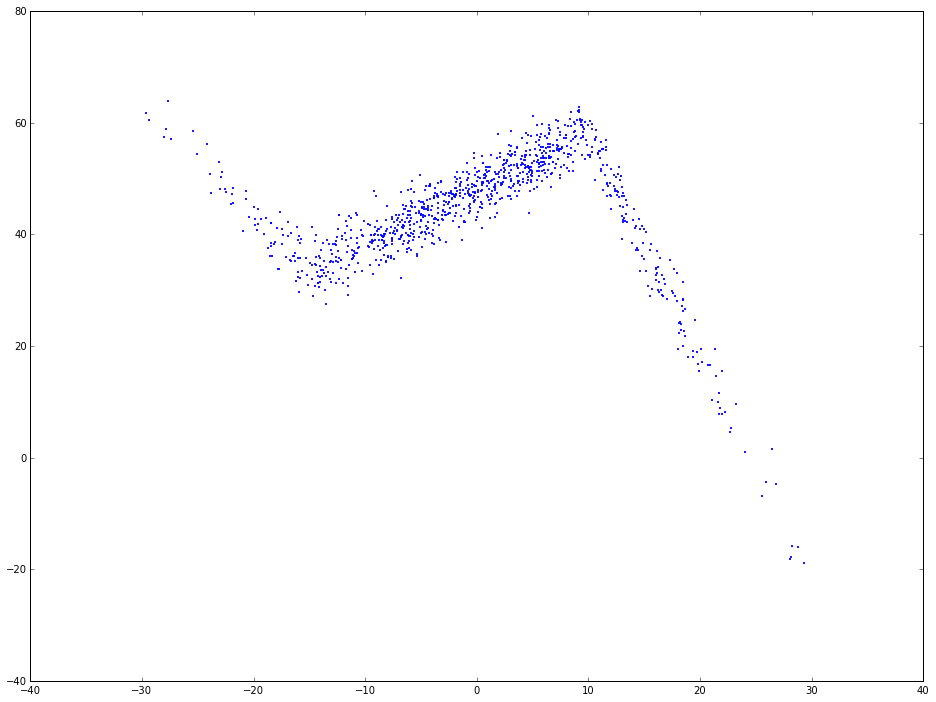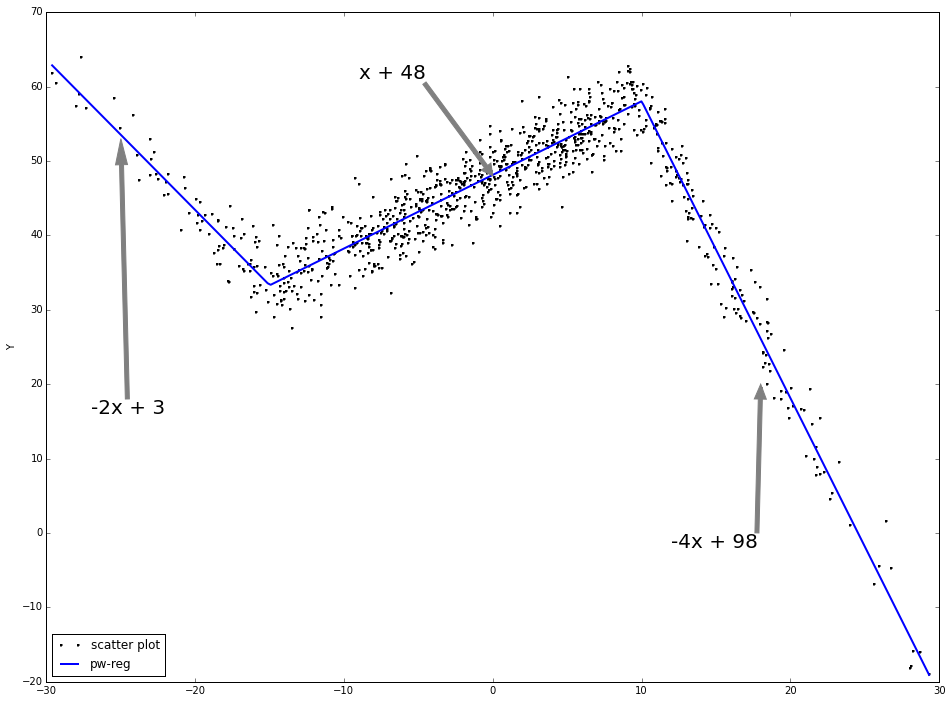# piecewise linear function and the explanation

Suppose the data is generated in this way: x is from random normal with mean 0, std = 10. length of x is 1000.

1. if x < -15, then y = -2 · x + 3

2. if -15 < x < 10, then y = x + 48

3. if x < 10, then y = -4 · x + 98

We can rewrite the above funcion in the following way:

$y = \alpha + \beta_1 \times x + \beta_2 \times (x + 15) I(x > -15) + \beta_3 \times (x - 10) I(x > 10)$

$y = 3 - 2 \times x + 3 \times (x + 15) I(x > -15) - 5 \times (x - 10) I(x > 10)$

python code to generate the simulation data. In different intervals of x, the relation between x and y is different.

import pandas as pd
import numpy as np
import matplotlib.pyplot as plt
import statsmodels.formula.api as smf

# plot inline rather than pop out
%matplotlib inline
# change the plot size, default is (6, 4) which is a little small
plt.rcParams['figure.figsize'] = (16, 12)

np.random.seed(9999)
x = np.random.normal(0, 1, 1000) * 10
y = np.where(x < -15, -2 * x + 3 , np.where(x < 10, x + 48, -4 * x + 98)) + np.random.normal(0, 3, 1000)
plt.scatter(x, y, s = 5, color = u'b', marker = '.', label = 'scatter plt')
plt.show()As you can see, the relation between x and y is not simplely linear. If use linear regression to fit this, the regression line will be like the following:

# linear regression
f1 = smf.ols(formula = 'y ~ x', data = dtest).fit()
dtest['f1_pred'] = f1.predict()
# print f1.summary()

fig = plt.figure()
fig.patch.set_alpha = 0.5

ax.plot(x, y, linestyle = '', color = 'k', linewidth = 0.25, markeredgecolor='none', marker = '.', label = r'scatter plot')
ax.plot(dtest.x, dtest.f1_pred, color = 'blue', linestyle = '-', linewidth = 1, markeredgecolor='none', marker = '', label = r'lin-reg')
ax.annotate('$y = 44.25 - 0.0645 x$', (10, 43), xytext = (0.5, 0.4), fontsize = 20, textcoords = 'axes fraction',  arrowprops = dict(facecolor = 'grey', color = 'grey'))
plt.show()Piecewise linear regression:

1. for each interval, a linear line will be fitted.

2. the fitting function is continuous at the change points.

As is shown, the piecewise linear regression fits the data much better than linear regression directly.

# piecewise linear data prepare
x1 = np.where(x > -15, x + 15, 0)
x2 = np.where(x > 10, x - 10, 0)
dtest = pd.DataFrame([y, x, x1, x2]).T
dtest.columns = ['y', 'x', 'x1', 'x2']

# piecewise linear regression
f2 = smf.ols(formula = 'y ~ x + x1 + x2', data = dtest).fit()
dtest['f2_pred'] = f2.predict()
# print f2.summary()

dtest.sort('x', inplace = True)

fig = plt.figure(figsize = (16, 12))

ax.plot(x, y, linestyle = '', color = 'k', linewidth = 0.25, markeredgecolor='none', marker = '.', label = r'scatter plot')
ax.plot(dtest.x, dtest.f2_pred, color = 'b', linestyle = '-', linewidth = 2, markeredgecolor='none', marker = '', label = r'pw-reg')

# pd.DataFrame([x, f2_pred]).to_excel(r'c:\test.xlsx')

ax.annotate('-2x + 3', (-25, 53), xytext = (-.95, 0.4), fontsize = 20, textcoords = 'axes fraction', arrowprops = dict(facecolor = 'grey', color = 'grey'))
ax.annotate('x + 48', (0, 48), xytext = (0.35, 0.9), fontsize = 20, textcoords = 'axes fraction', arrowprops = dict(facecolor = 'grey', color = 'grey'))
ax.annotate('-4x + 98', (18, 20), xytext = (0.7, 0.2), fontsize = 20, textcoords = 'axes fraction', arrowprops = dict(facecolor = 'grey', color = 'grey'))

plt.legend(loc = 3, ncol = 1)

plt.show()# Using np.piecewise

np.piecewise will evaluate a piecewise-defined function. After the piecewise linear function is defined, we can use optimize.curve_fit to find the optimized solution to the parameters. The benefit is you don't need to define the cutoff point. It will automatically solve the function: finding both the coefficients and the cutoff points.

from scipy import optimize

def piecewise_linear(x, x0, x1, b, k1, k2, k3):
condlist = [x < x0, (x >= x0) & (x < x1), x >= x1]
funclist = [lambda x: k1*x + b, lambda x: k1*x + b + k2*(x-x0), lambda x: k1*x + b + k2*(x-x0) + k3*(x - x1)]
return np.piecewise(x, condlist, funclist)

p , e = optimize.curve_fit(piecewise_linear, x, y)

xd = np.linspace(-30, 30, 1000)
plt.plot(x, y, "o")
plt.plot(xd, piecewise_linear(xd, *p))